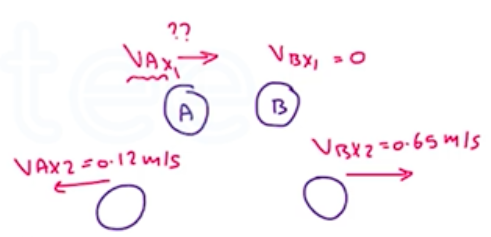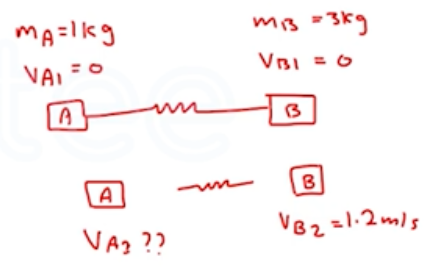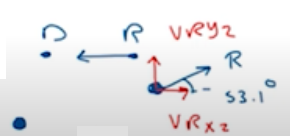Need Help?

Subscribe to Physics 1

###### \${selected_topic_name}
• Notes

$\begin{array}{l}{\text { On a frictionless, horizontal air table, puck } A \text { (with mass }} \\ {0.250 \mathrm{kg} \text { is moving toward puck } B(\text { with mass } 0.350 \mathrm{kg}), \text { which is }} \\ {\text { initially at rest. After the collision, puck } A \text { has a velocity of }} \\ {0.120 \mathrm{m} / \mathrm{s} \text { to the left, and puck } B \text { has a velocity of } 0.650 \mathrm{m} / \mathrm{s} \text { to }} \\ {\text { the right. (a) What was the speed of puck } A \text { before the collision? }} \\ {\text { (b) Calculate the change in the total kinetic energy of the system }} \\ {\text { that occurs during the collision. }}\end{array}$$P_{1x}=P_{2 x}$

$m_{A} v_{A{x{1}}}+m_{B} v_{B x1}=m_{A} v_{A x{2}}+m_{B} v_{B x{2}}$

$(0.25) v_{ A x1}=-(0.25)(0.12)+0.35(0.65)$

$∴ V_{A X 1}=\frac{-0.25(0.12)+0.35(0.65)}{0.25}=0.79 \mathrm{m/s}$

$\Delta k=k_{2}-k_{1} \quad \longrightarrow k_{1}=\frac{1}{2} m_{A} v_{A x 1}+\frac{1}{2} m_B v_{BX1}=\frac{1}{2}(0.25)(0.79)=0.078 \mathrm{J}$

$k_{2}=\frac{1}{2} m_{A} v_{AX2}+\frac{1}{2} m_{B} v_{Bx2}=\frac{1}{2}(0.25)(0.12)+\frac{1}{2}(0.35)(0.65)$

$=0.0757 J$

$\Delta k=k_{2}-k_{1}=0.0757-0.078=-0.0023 \mathrm{J}$

$\begin{array}{l}{\text { Block } A \text { in Fig. E8.24 has mass } 1.00 \mathrm{kg} \text { , and block } B \text { has }} \\ {\text { mass } 3.00 \mathrm{kg} \text { . The blocks are forced together, compressing a spring }} \\ {S \text { between them; then the system is released from rest on a level, }} \\ {\text { frictionless surface. The spring, which has negligible mass, is not }} \\ {\text { fastened to either block and drops to the surface after it has }} \\ {\text { expanded. Block } B \text { acquires a speed of } 1.20 \mathrm{m} / \mathrm{s} \text { . (a) What is the }} \\ {\text { final speed of block } A ? \text { (b) How much potential energy was stored }} \\ {\text { in the compressed spring? }}\end{array}$$P_{1}=P_{2}=m_{A} v_{A 1}+m_{B} v_{B 1}$

$P_{2}=m_{A} v_{A 2}+m_{B} v_{B 2}=0$

$m_{A} v_{A{2}}=-m_{B} v_{B{2}}$

$(1) V_{A 2}=-3(1.2) \rightarrow V_{A 2}=\frac{-3(1.2)}{1}=-3.6 \mathrm{m} / \mathrm{s}$

$k_{1}+U_{1}+W_{\text {others }}=k_{2}+U_{2}$

$U_{1}=k_{2} \quad \longrightarrow \quad k_{2}=\frac{1}{2} m_{A{2}} {v_{A2}}^{2}+\frac{1}{2} m_{B} {v_{B}}^{2}$

$=\frac{1}{2}(1)(-3.6)^{2}+\frac{1}{2}(3)(1.2)^{2}=8.64 \mathrm{J}$

$U=8.64 \mathrm{J}$

$\begin{array}{l}{\text { Two ice skaters, Daniel (mass } 65.0 \mathrm{kg}) \text { and Rebecca }} \\ {\text { (mass } 45.0 \mathrm{kg}), \text { are practicing. Daniel stops to tie his shoelace and, }} \\ {\text { while at rest, is struck by Rebecca, who is moving at } 13.0 \mathrm{m} / \mathrm{s}} \\ {\text { before she collides with him. After the collision, Rebecca has a }} \\ {\text { velocity of magnitude } 8.00 \mathrm{m} / \mathrm{s} \text { at an angle of } 53.1^{\circ} \text { from her }} \\ {\text { initial direction. Both skaters move on the frictionless, horizon- }}\end{array}$

$\begin{array}{l}{\text { tal surface of the rink. (a) What are the magnitude and direction }} \\ {\text { of Daniel's velocity after the collision? (b) What is the change }} \\ {\text { in total kinetic energy of the two skaters as a result of the }} \\ {\text { collision? }}\end{array}$

$m_{D}=65 k g \quad m_{R}=45 k g$

$v_{D{1}=0} \quad v_{R{1} x}=13 \mathrm{m} / \mathrm{s}$

$V_{D{2}} ? ? \quad V_{R{2x}}=8 m /s \quad \angle \theta=53.1^{\circ}$$V_{R y 2}=V_{ R2} \sin \theta=8 \sin 53.1=6.4 \mathrm{m/s}$

$V _{Rx 2}=V _{R2} \cos \theta=8 \cos 53.1=4.8 \mathrm{m/s}$

(x)

$P_{1x} =P_{2x}$

$m_ R V_{R{1}}+m_{D} V_{D 1}=m_{R} V_{Rx2}+m_{D} V_{Dx 2}$

$V_{Dx2}=\frac{M_{R} V_{R1}-m_{R} v_{R{x}}}{m_{D}}$

(y)

$R_{1y}=P_{2y}$

$m_R V_{Ry1}+m_D V_{Dy1}=m_R V_{Ry2}+m_D V_{Dy2}$

$V_{D y{2}}=\frac{-m_{R} v_{R y{2}}}{m_{D}}=-4.43 \mathrm{m} / \mathrm{s}$

$V_{D{2}}=\sqrt{(5.68)^{2}+(4.43)^{2}}=7.2 \mathrm{m} / \mathrm{s}$

$\tan \theta=\frac{v _{D2y}}{v_{D 2 x}}=\theta=38^{\circ}$

$\Delta K=K_{2}-K_{1}$

${k_{1}=\frac{1}{2} m_{R} {v_{R1}}^{2}+0=\frac{1}{2}(45)(13)^{2}=3 \cdot 8 \times 10^{3} J}$

$k_{2}=\frac{1}{2} m_{R} {v_{R2}}^{2}+\frac{1}{2} m_{D} {v_{D2}}^{2}=\frac{1}{2}(45)(8)^{2}+\frac{1}{2}(65)(7.2)^{2}=3.12 *10^{3} \mathrm{J}$

$\Delta k=3.12 \times 10^{3}-3.8 \times 10^{2}=-680 \mathrm{J}$